# 9 - Enemies and Basic AI

What would a dungeon game be without enemies? Let's add some!

1. This should be second nature by now - add two new entity types in your Ogmo project, `enemy` and `boss`: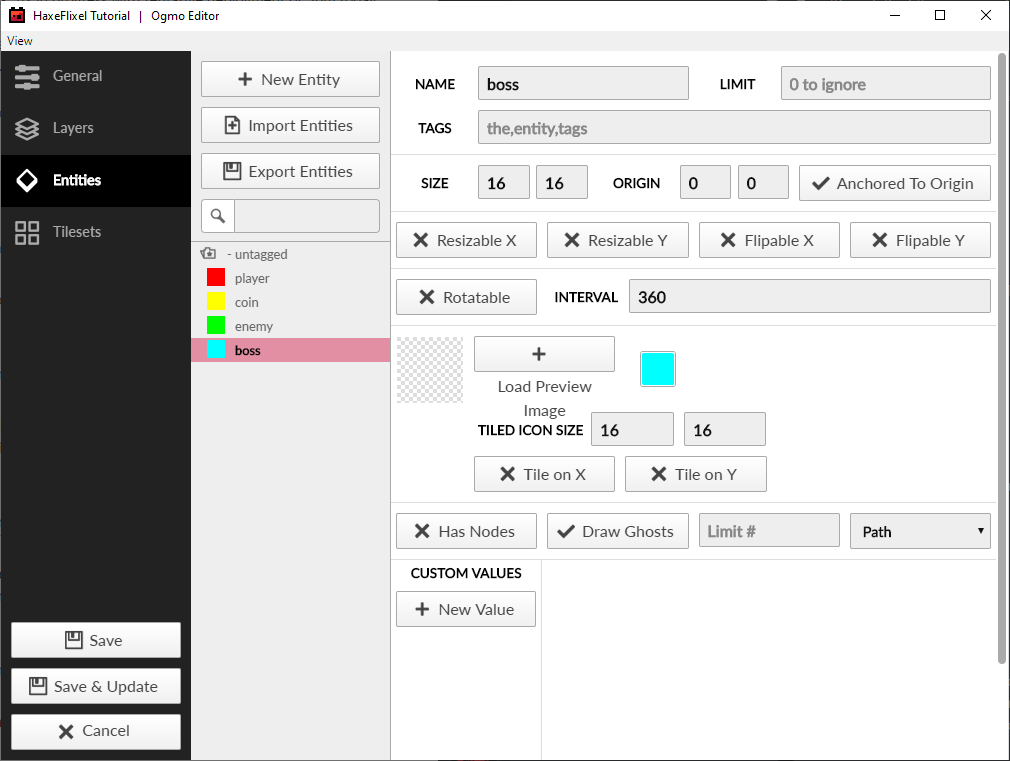2. Then scatter some enemies and a boss around the map.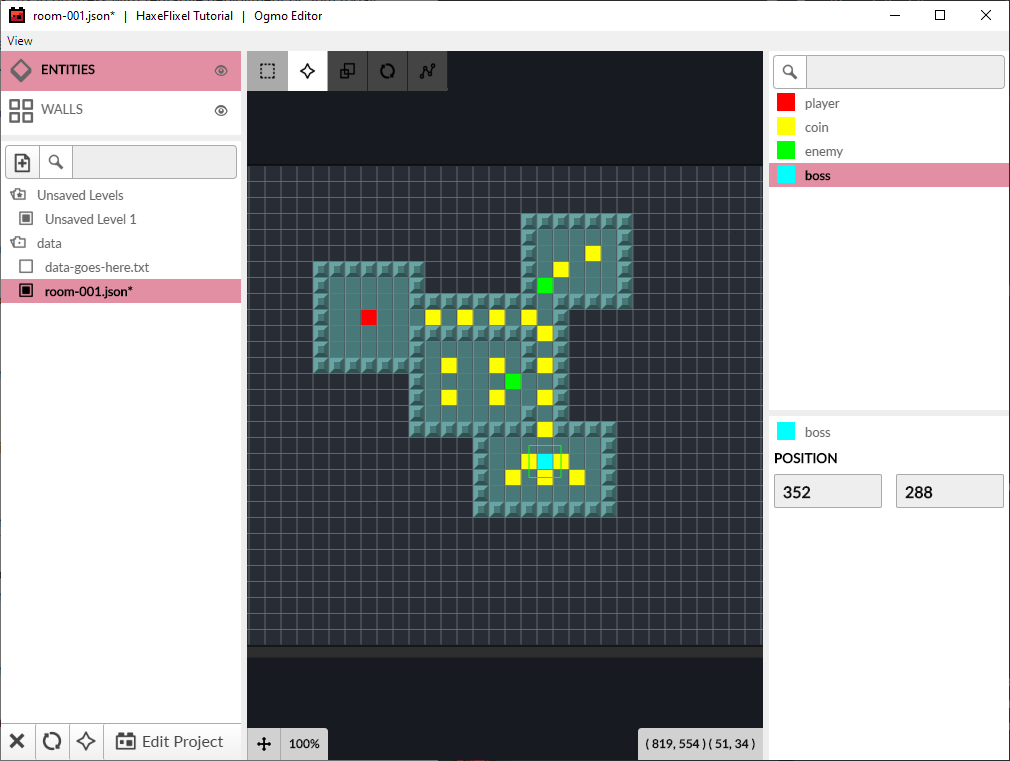3. So we want to have 2 different enemies in our game. We'll need spritesheets for both of them, with 16x16 pixel frames and the same animation frames as our player. Name them `enemy.png` and `boss.png` and put them in the `assets/images` folder. You can use these, if you want (thanks, again, Vicky!):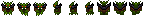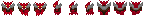Note: make sure that your enemy sprites are functionally the same - they should have the same number of frames for each `facing` animation.

4. Let's add a new some code for enemies. Since we're going to have two different types of enemies, regular enemies and the boss, let's start by creating an `EnemyType` enumeration:

`````` enum EnemyType
{
REGULAR;
BOSS;
}
``````

This basically just gives us two handy constants that we can use to distuingish them. Our `Enemy` class is going to look a lot like our `Player`:

`````` package;

import flixel.FlxObject;
import flixel.FlxSprite;

class Enemy extends FlxSprite
{
static inline var SPEED:Float = 140;

var type:EnemyType;

public function new(x:Float, y:Float, type:EnemyType)
{
super(x, y);
this.type = type;
var graphic = if (type == BOSS) AssetPaths.boss__png else AssetPaths.enemy__png;
setFacingFlip(FlxObject.LEFT, false, false);
setFacingFlip(FlxObject.RIGHT, true, false);
animation.add("d", [0, 1, 0, 2], 6, false);
animation.add("lr", [3, 4, 3, 5], 6, false);
animation.add("u", [6, 7, 6, 8], 6, false);
drag.x = drag.y = 10;
width = 8;
height = 14;
offset.x = 4;
offset.y = 2;
}

override public function update(elapsed:Float)
{
if ((velocity.x != 0 || velocity.y != 0) && touching == FlxObject.NONE)
{
if (Math.abs(velocity.x) > Math.abs(velocity.y))
{
if (velocity.x < 0)
facing = FlxObject.LEFT;
else
facing = FlxObject.RIGHT;
}
else
{
if (velocity.y < 0)
facing = FlxObject.UP;
else
facing = FlxObject.DOWN;
}

switch (facing)
{
case FlxObject.LEFT, FlxObject.RIGHT:
animation.play("lr");

case FlxObject.UP:
animation.play("u");

case FlxObject.DOWN:
animation.play("d");
}
}
super.update(elapsed);
}
}
``````

The main difference is that we have a new `type` variable, which we will use to figure out which enemy sprite to load, and which one we're dealing with, etc.

5. Next, we'll make a `FlxGroup` in our `PlayState` to hold our enemies, and load them into the map, very much the same way we did our coins.

At the top of our class, add:

`````` var enemies:FlxTypedGroup<Enemy>;
``````

In the create function, right after we add our coin group:

`````` enemies = new FlxTypedGroup<Enemy>();
``````

We will also need to add two more cases to our `placeEntities()` function:

`````` else if (entity.name == "enemy")
{
enemies.add(new Enemy(entity.x + 4, entity.y, 0));
}
else if (entity.name == "boss")
{
enemies.add(new Enemy(entity.x + 4, entity.y, 1));
}
``````

6. (optional step) Our `placeEntities()` is starting to get a bit repetitive. Each `if` checks `entity.name`, and each time we use `entity.x` and `entity.y`.

Let's fix this by using a `switch-case` instead of an `if`/`else`-chain, as well as adding some temporary `x` and `y` variables:

`````` var x = entity.x;
var y = entity.y;

switch (entity.name)
{
case "player":
player.setPosition(x, y);

case "coin":
coins.add(new Coin(x + 4, y + 4));

case "enemy":
enemies.add(new Enemy(x + 4, y, REGULAR));

case "boss":
enemies.add(new Enemy(x + 4, y, BOSS));
}
``````

There, that's a lot easier to read!

Now let's give our enemies some brains.

In order to let our enemies 'think', we're going to utilize a very simple Finite-state Machine (FSM). Basically, the FSM works by saying that a given machine (or entity) can only be in one state at a time. For our enemies, we're going to give them 2 possible states: `Idle` and `Chase`. When they can't 'see' the player, they will be `Idle` - wandering around aimlessly. Once the player is in view, however, they will switch to the `Chase` state and run towards the player.

1. Shouldn't be that hard! First, we'll make our `FSM` class:

`````` class FSM
{
public var activeState:Float->Void;

public function new(initialState:Float->Void)
{
activeState = initialState;
}

public function update(elapsed:Float)
{
activeState(elapsed);
}
}
``````
2. Next, we'll change our `Enemy` class a little.

We need to define these variables at the top of the class:

`````` var brain:FSM;
var idleTimer:Float;
var moveDirection:Float;
var seesPlayer:Bool;
var playerPosition:FlxPoint;
``````
3. At the end of the constructor, add:

`````` brain = new FSM(idle);
idleTimer = 0;
playerPosition = FlxPoint.get();
``````
4. And then add the following functions:

`````` function idle(elapsed:Float)
{
if (seesPlayer)
{
brain.activeState = chase;
}
else if (idleTimer <= 0)
{
if (FlxG.random.bool(1))
{
moveDirection = -1;
velocity.x = velocity.y = 0;
}
else
{
moveDirection = FlxG.random.int(0, 8) * 45;

velocity.set(SPEED * 0.5, 0);
velocity.rotate(FlxPoint.weak(), moveDirection);

}
idleTimer = FlxG.random.int(1, 4);
}
else
idleTimer -= elapsed;

}

function chase(elapsed:Float)
{
if (!seesPlayer)
{
brain.activeState = idle;
}
else
{
FlxVelocity.moveTowardsPoint(this, playerPosition, Std.int(SPEED));
}
}
``````

Also add this line to `update()` before `super.elapsed()`:

`````` brain.update(elapsed);
``````

The way this is going to work is that each enemy will start in the `Idle` state. In the `PlayState` we will have each enemy check to see if it can see the player or not. If it can, it will switch to the `Chase` state, until it can't see the player anymore. While in the `Idle` state, every so often (in random intervals) it will choose a random direction to move in for a little while (with a small chance to just stand still). While in the `Chase` state, they will move directly towards the player.

5. Let's jump over to the `PlayState` to add our player's vision logic. In `update()`, under the overlap and collision checks, add:

`````` FlxG.collide(enemies, walls);
enemies.forEachAlive(checkEnemyVision);
``````
6. Next, add the `checkEnemyVision()` function:

`````` function checkEnemyVision(enemy:Enemy)
{
if (walls.ray(enemy.getMidpoint(), player.getMidpoint()))
{
enemy.seesPlayer = true;
enemy.playerPosition.copyFrom(player.getMidpoint());
}
else
{
enemy.seesPlayer = false;
}
}
``````

That's all there is to it! Try out your game and make sure it works.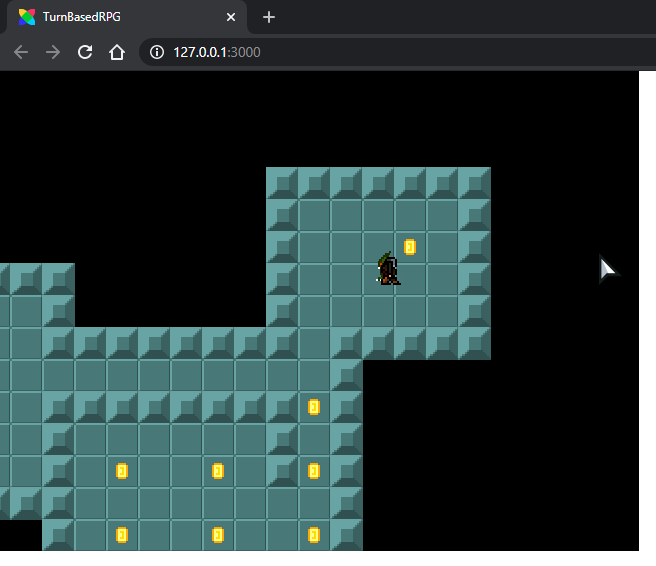Next, we'll add some UI to the game, and add our RPG-style combat so you can fight the enemies!